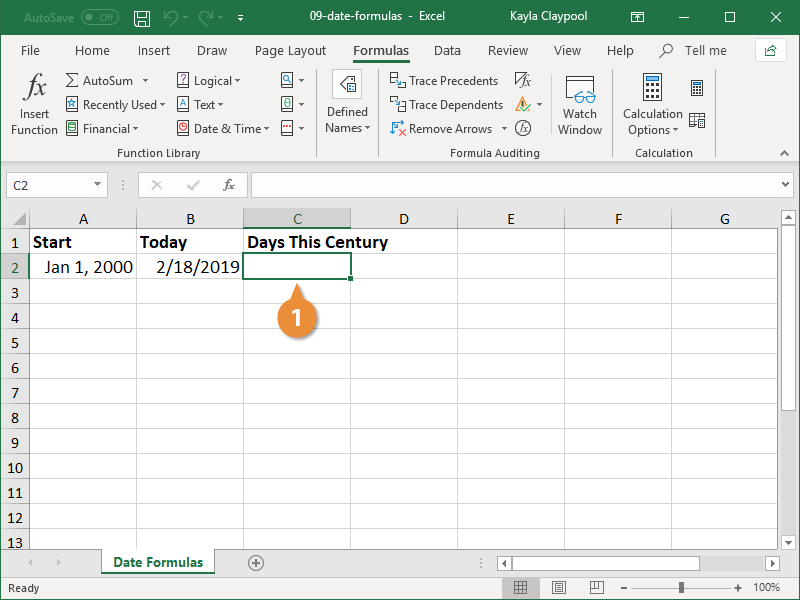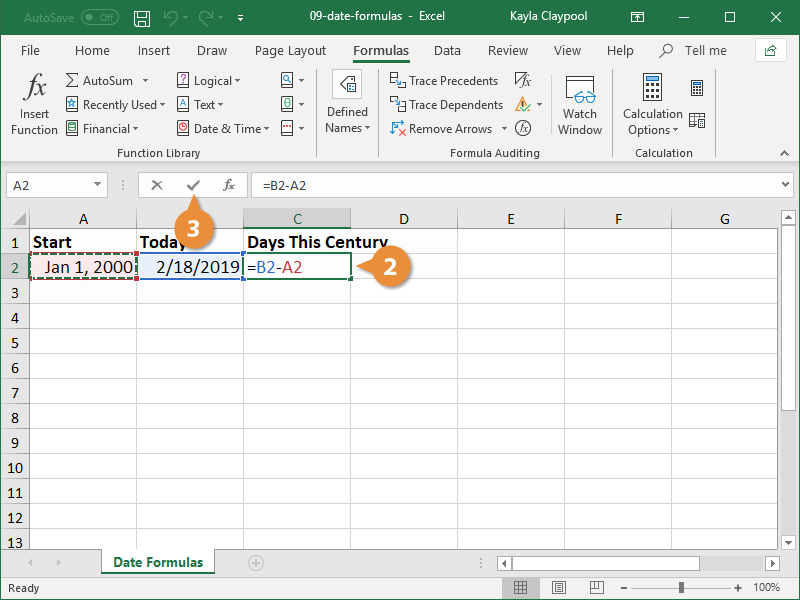Excel Date Functions | CustomGuide# Excel Date Functions

How to Use Date Formulas in Excel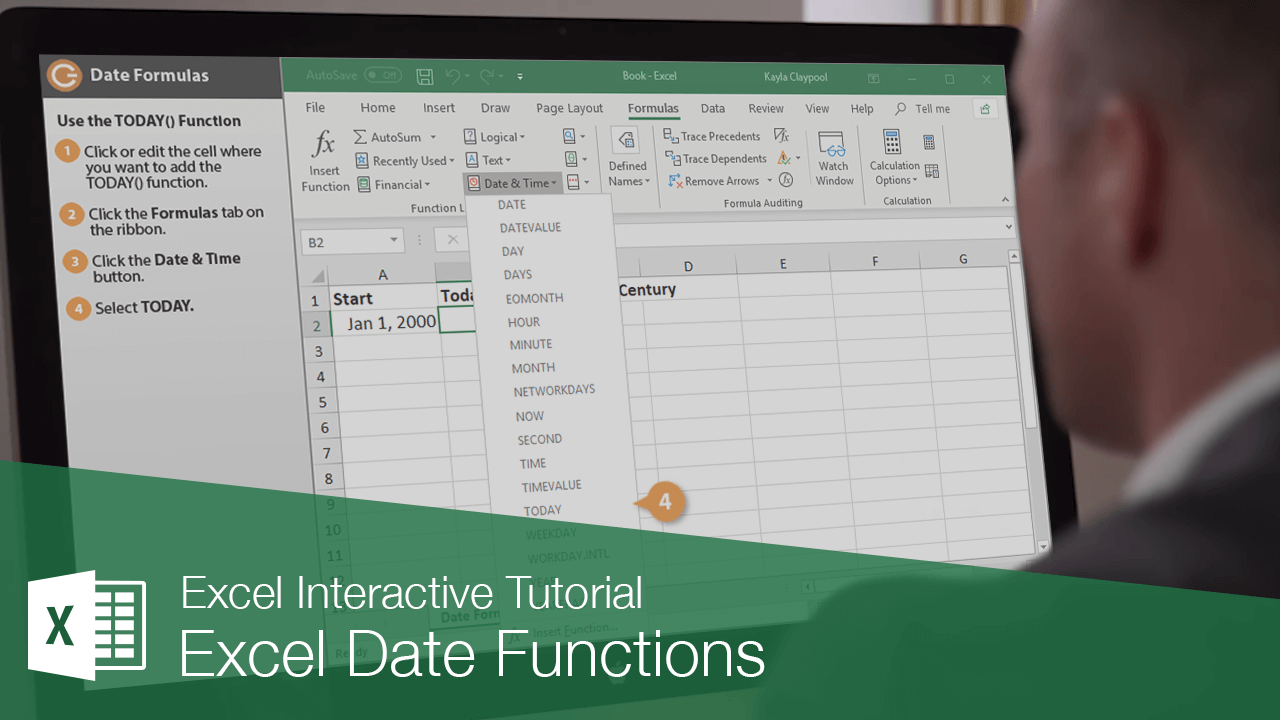You can use dates and time in your formulas just like any other value. For example, if cell A1 contained the entry 5/1/19 you could use the formula =A1+100 to calculate the date 100 days later, which is 8/9/19.

One very important thing to know about working with date and time functions: while Excel can display dates and times using just about any format, it stores dates as chronological numbers called serial values. So, when you think of dates as months, days, and years, such as May 1, 2019, Excel thinks of dates in terms of serial numbers, such as 36281.

Since the date and time formulas often return serial number values, you should format any cells with date or time formulas with date and time formats that you can easily understand.

### Common Date Functions

This table lists some of the date and time functions available in Excel.

Function Name Function What the Function Does
Date =DATE(year, month, day) Enters a date in the cell. Example: DATE(2019,5,1) equals May 1, 2019.
Today =TODAY( ) A special version of the DATE function. The DATE function returns the value of any date; the TODAY function returns the value of the current date.
Time =TIME(hour, minute, second) Enters a time in the cell. Uses a 24-hour (military) time system.
Example: TIME(14,30,0) equals 2:30 PM.
Now =NOW( ) A special version of the TIME function. The TIME function returns the value of any time; the NOW function returns the value of the current time.
Year =YEAR(serial_number, return_type) Returns a value of the year for a specific date. The serial_number argument is a date value (or reference to one).
Example: YEAR("3/15/2019") equals 2019.
Month =MONTH(serial_number, return_type) Returns a value of the month for a specific date. The serial_number argument is a date value (or reference to one).
Example: MONTH("3/15/2019") equals 3.
Day =DAY(serial_number, return_type) Returns a value of the day for a specific date. The serial_number argument is a date value (or reference to one). Example: DAY("3/15/2019") equals 15.

### TODAY() Function

If you need to calculate values based on dates, a very helpful function is TODAY, which returns today’s date.

1. Select the cell where you want to add the TODAY() function.
2. Click the Formulas tab on the ribbon.
3. Click the Date & Time button.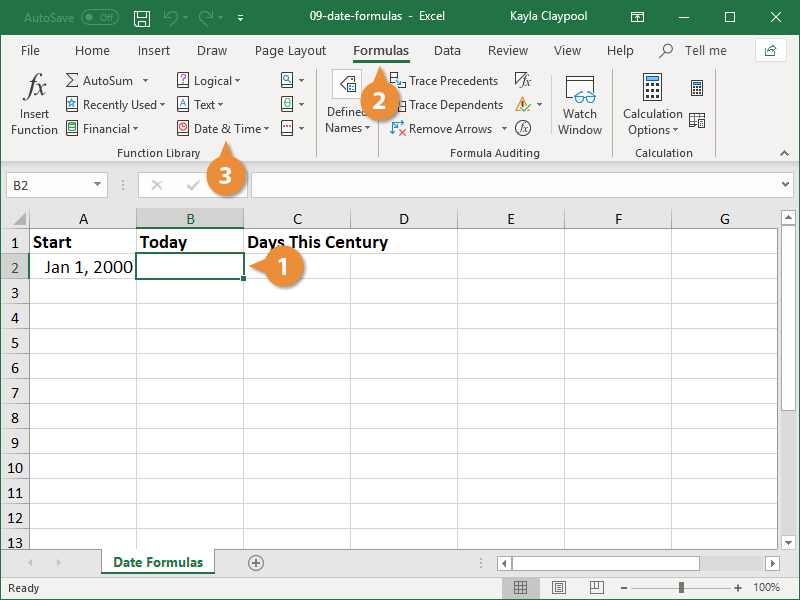4. Select Today.
5. Click OK.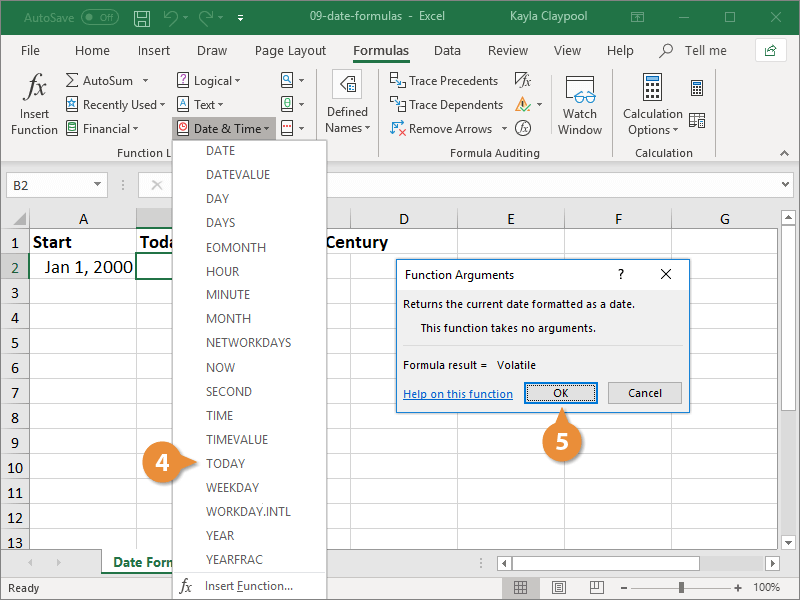You can also insert the function by typing =TODAY() in the cell.